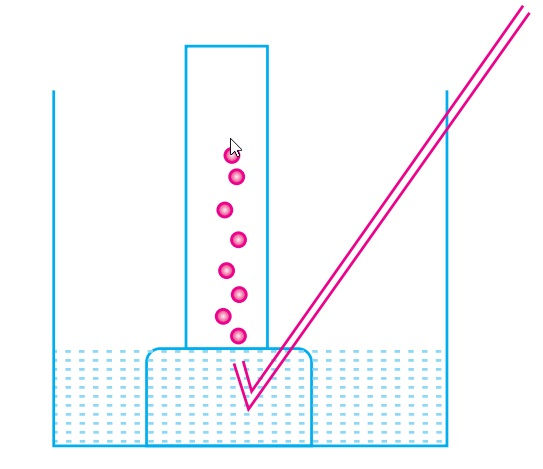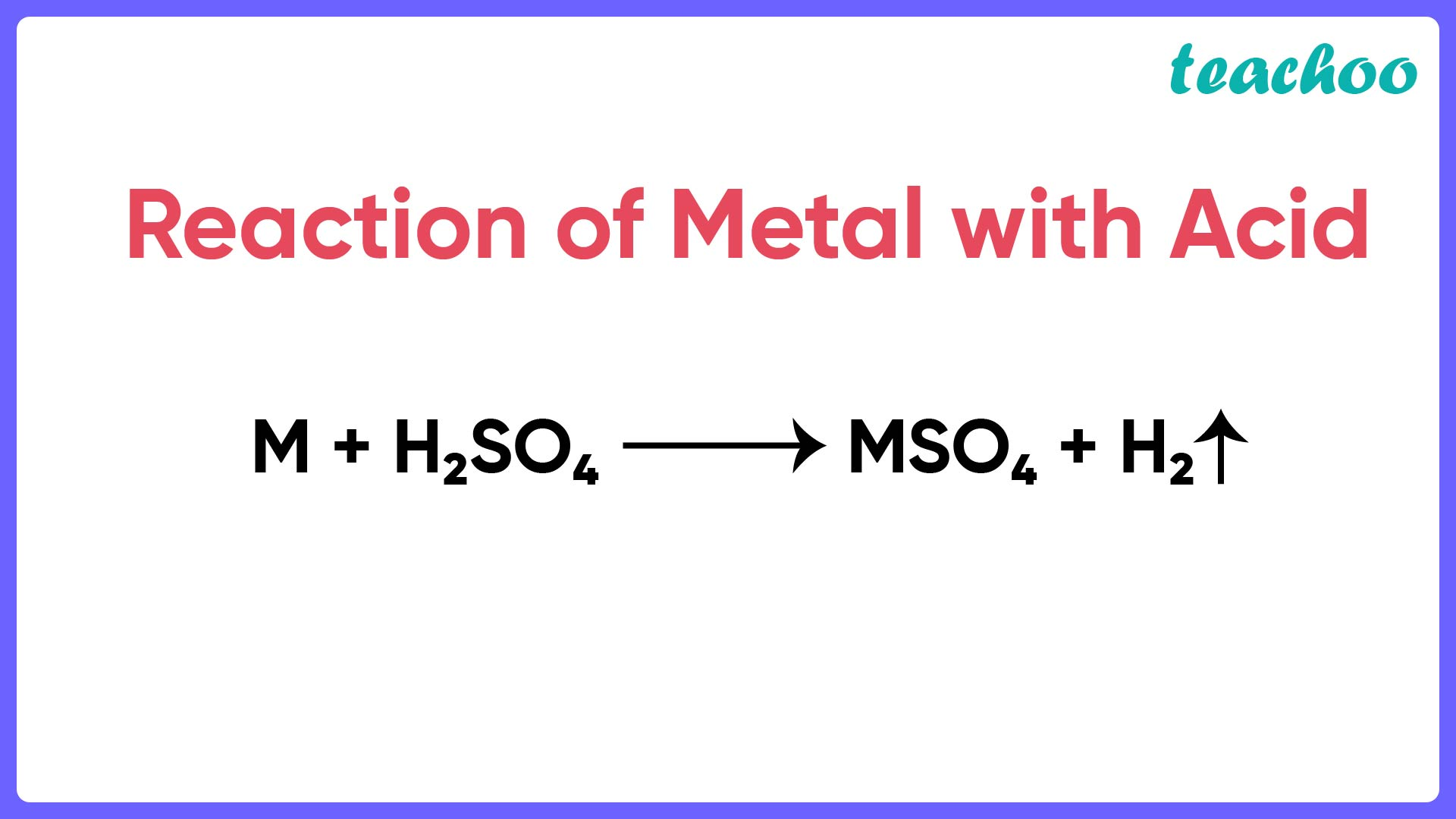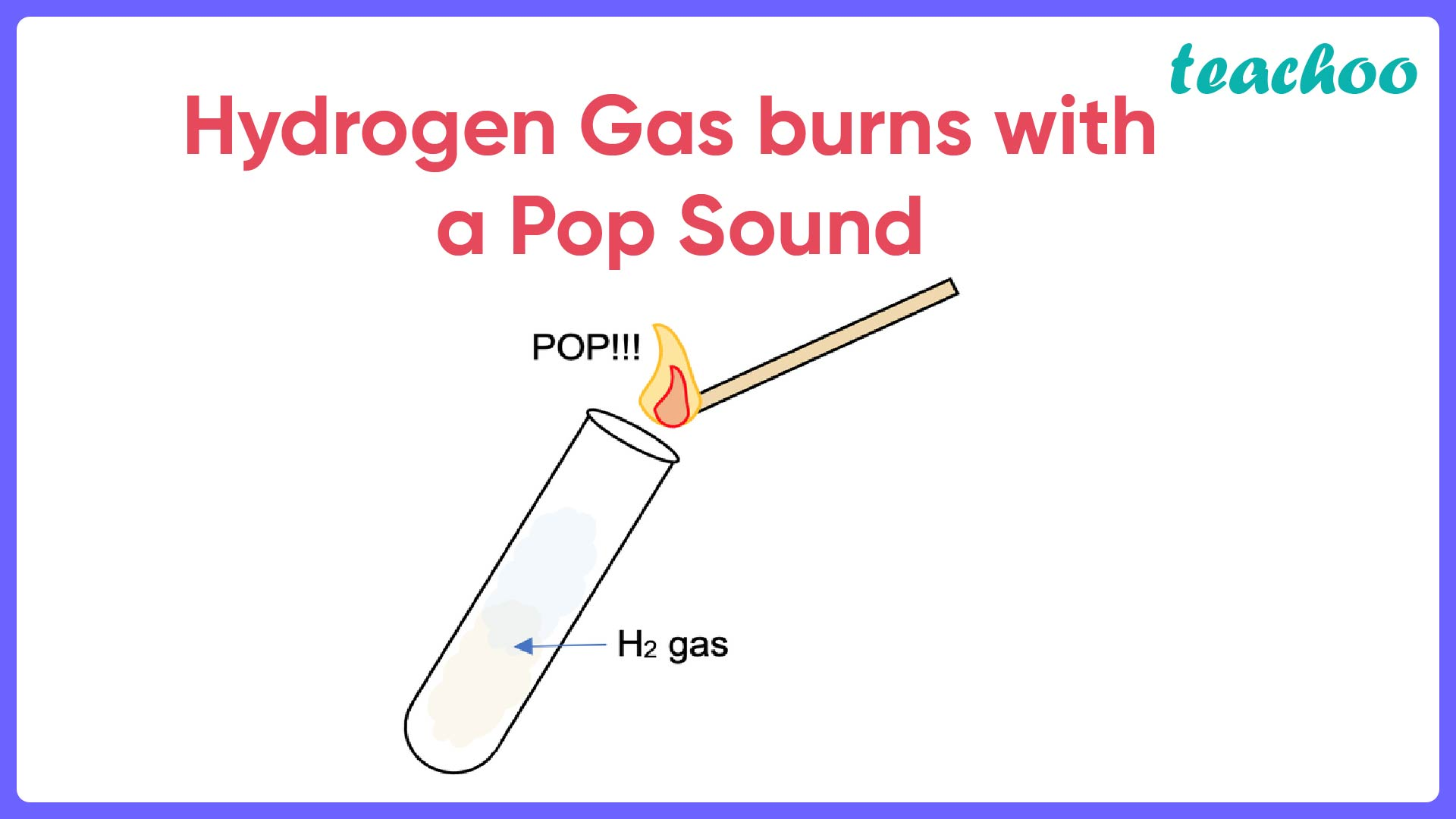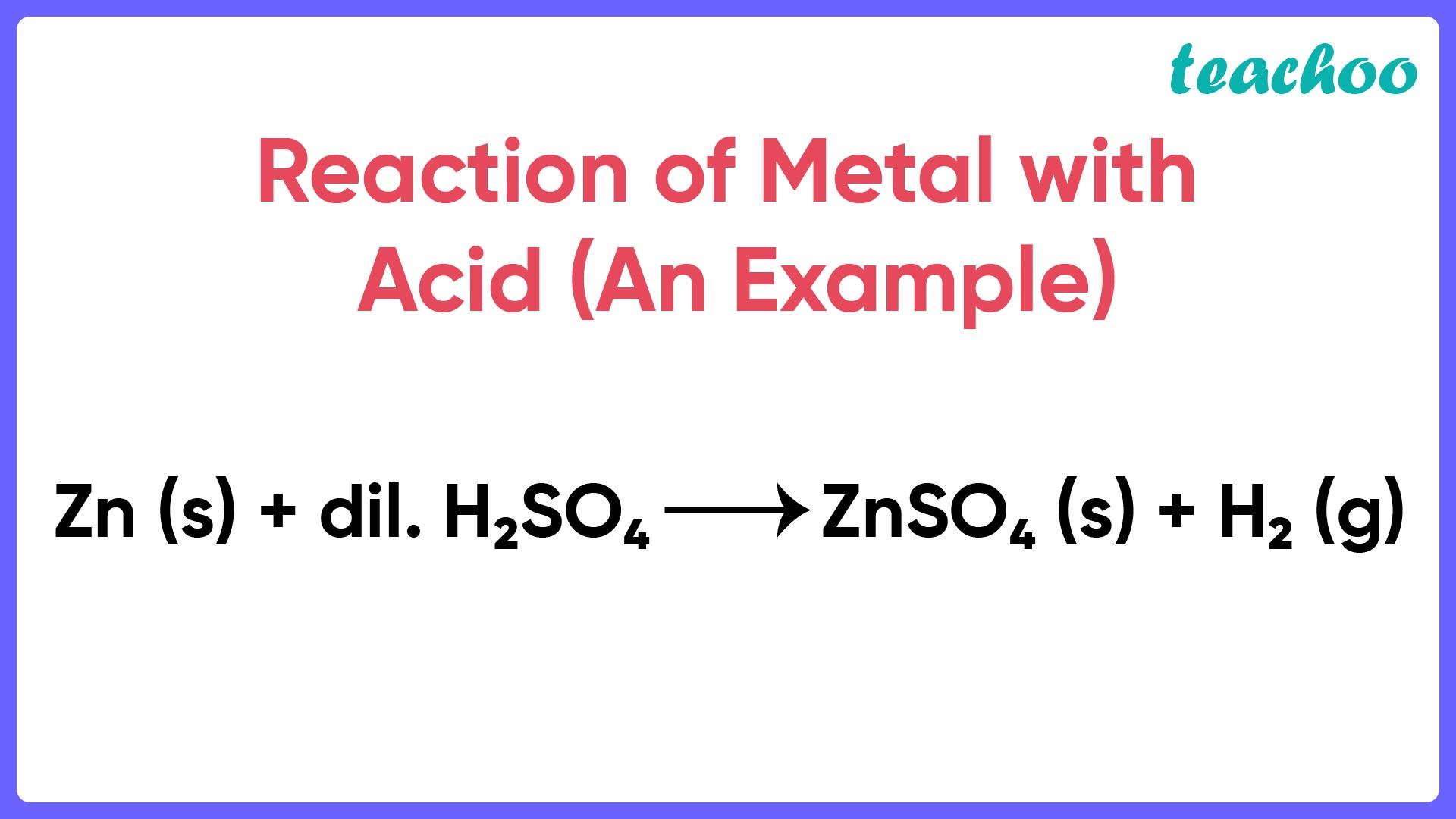Case Based Questions (MCQ)

Class 10
Chapter 2 Class 10 - Acids, Bases and Salts (Term 1)

## A metal is treated with dilute sulphuric acid. The  gas evolved is collected by the method shown in  the figure:## (iv) Carbon dioxide

Ans.

When a metal is reacted with dilute sulphuric acid, hydrogen gas is evolved.

The reaction is as follows:

M + H 2 SO 4   →MSO 4 + H 2So, the correct answer is (i) Hydrogen gas.

## ( b) Is the gas lighter or heavier than air? How will  you test the gas?

Ans.

Hydrogen gas is lighter than air

Test for presence of hydrogen gas:

• When a burning splinter is brought near the gas, it burns with a pop sound.
• This pop sound is produced due to the burning of hydrogen gas.## (c) If the metal used above is zinc then write the  chemical equation for the evolution of gas.

Ans.

The reaction is as follows:

Zn(s) + H 2 SO 4 (dil) → ZnSO 4 (aq) + H 2 (g)↑## (iv) all of the above

Ans.

Liquid hydrogen is used as a fuel in rockets.

So, the correct answer is (ii) Liquid